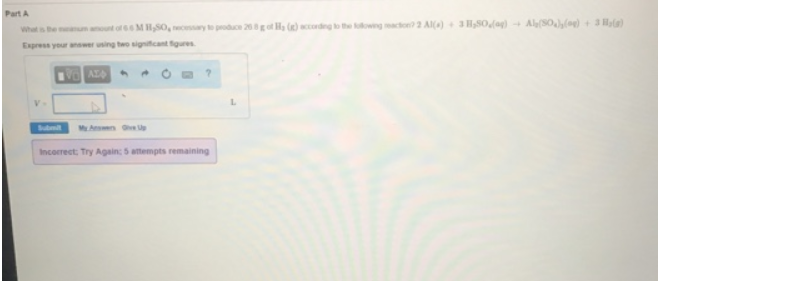# Problem: What is the minimum amount of 6.6 M H2SO4 to produce 26.8 g of H2 (g) according to the following reaction? 2Al (s) + 3H2SO4 (aq) → Al2(SO4)2 (aq) + 3H2 (s) Express your answer using two significance figures.

###### FREE Expert Solution
82% (205 ratings)###### Problem Details

What is the minimum amount of 6.6 M H2SO4 to produce 26.8 g of H2 (g) according to the following reaction?

2Al (s) + 3H2SO4 (aq) → Al2(SO4)(aq) + 3H2 (s)